### GAN的来源

14年Goodfellow提出Generative adversarial nets即生成式对抗网络，它要解决的问题是如何从训练样本中学习出新样本，训练样本是图片就生成新图片，训练样本是文章就输出新文章等等。

GANs简单的想法就是用两个模型， 一个生成模型，一个判别模型。判别模型用于判断一个给定的图片是不是真实的图片（从数据集里获取的图片），生成模型的任务是去创造一个看起来像真的图片一样的图片，有点拗口，就是说模型自己去产生一个图片，可以和你想要的图片很像。而在开始的时候这两个模型都是没有经过训练的，这两个模型一起对抗训练，生成模型产生一张图片去欺骗判别模型，然后判别模型去判断这张图片是真是假，最终在这两个模型训练的过程中，两个模型的能力越来越强，最终达到稳态。

### GAN的基本组成

GAN 模型中的两位博弈方分别由生成式模型（generative model）和判别式模型（discriminative model）充当。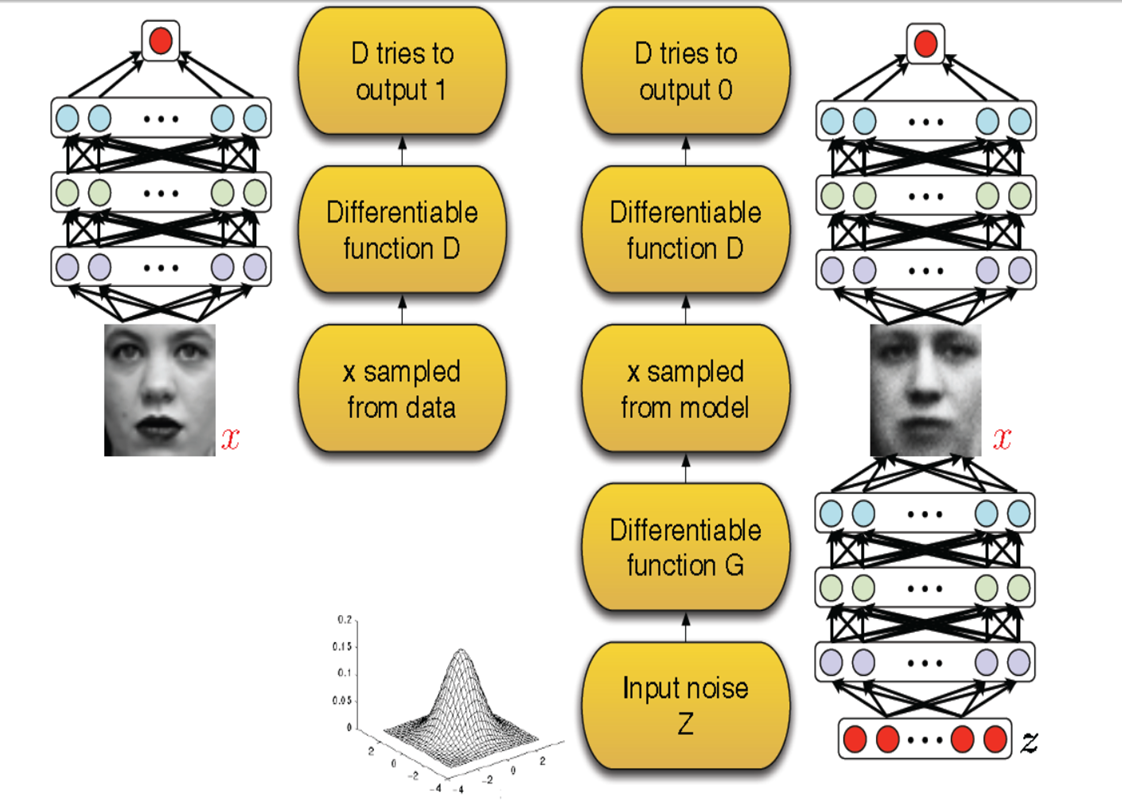GAN的训练过程可以看成一个博弈的过程，也可以看成2个人在玩一个极大极小值游戏，可以用如下公式表示：

$$\min \limits_{G}\max\limits_{D}GAN(D,G)=E_{x\sim P_{data}(x)}[logD(x)]+E_{z\sim p_z(z)}[log(1-D(G(z)))]$$

$$\max\limits_{D}GAN(D,G)=E_{x\sim P_{data}(x)}[logD(x)]+E_{z\sim p_z(z)}[log(1-D(G(z)))]$$

$$\min\limits_{G}GAN(D,G)=E_{z\sim p_z(z)}[log(1-D(G(z)))]$$

### GAN的训练过程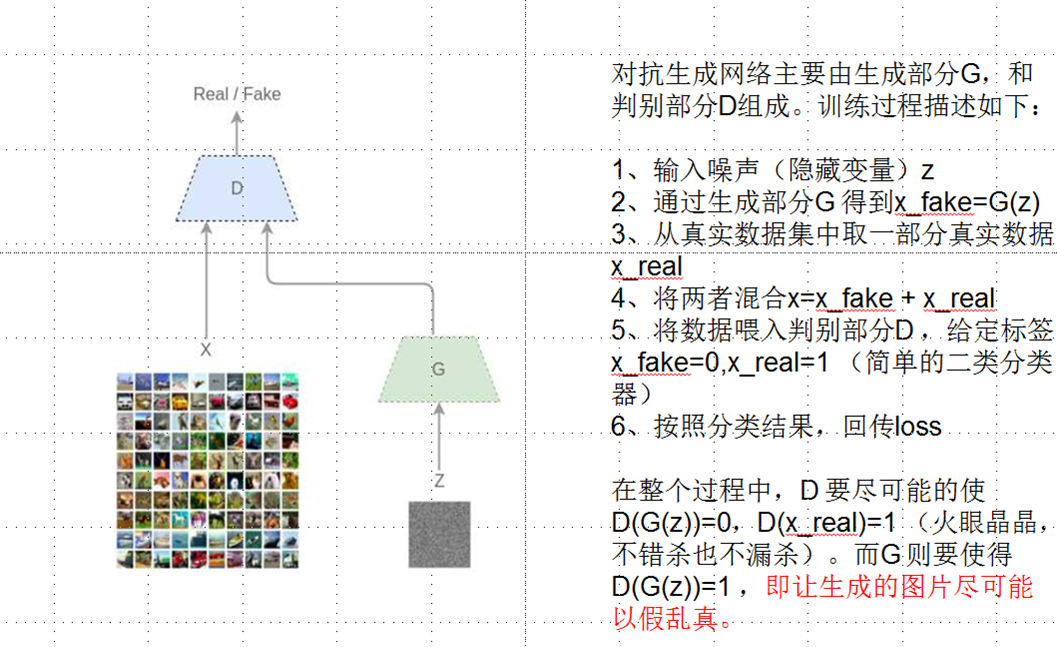GAN的算法流程和动态求解过程如下图所示：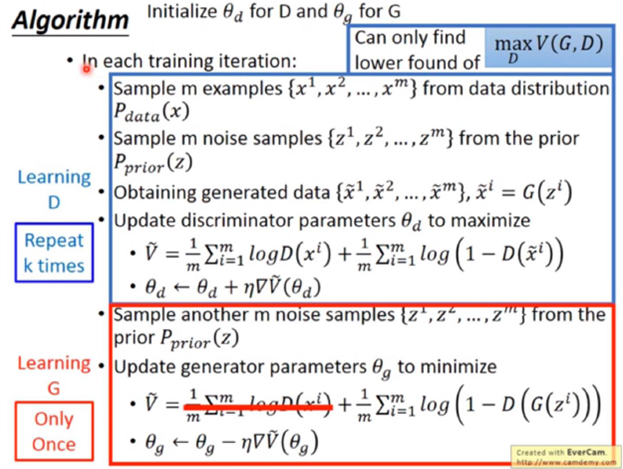### GAN的网络结构

#### 判别器(卷积)$$o=\lfloor\frac{i+2p-k}{s}\rfloor+1$$

#### 生成器(反卷积)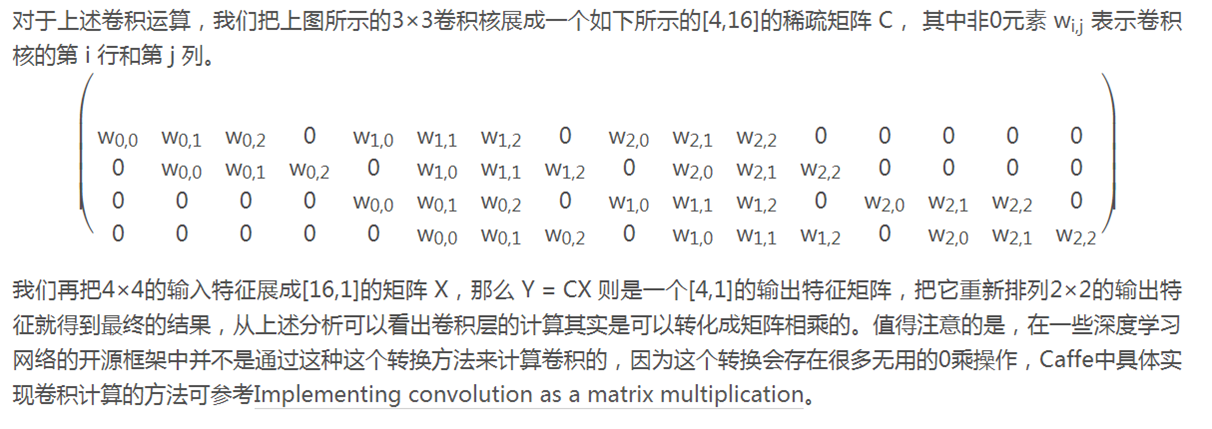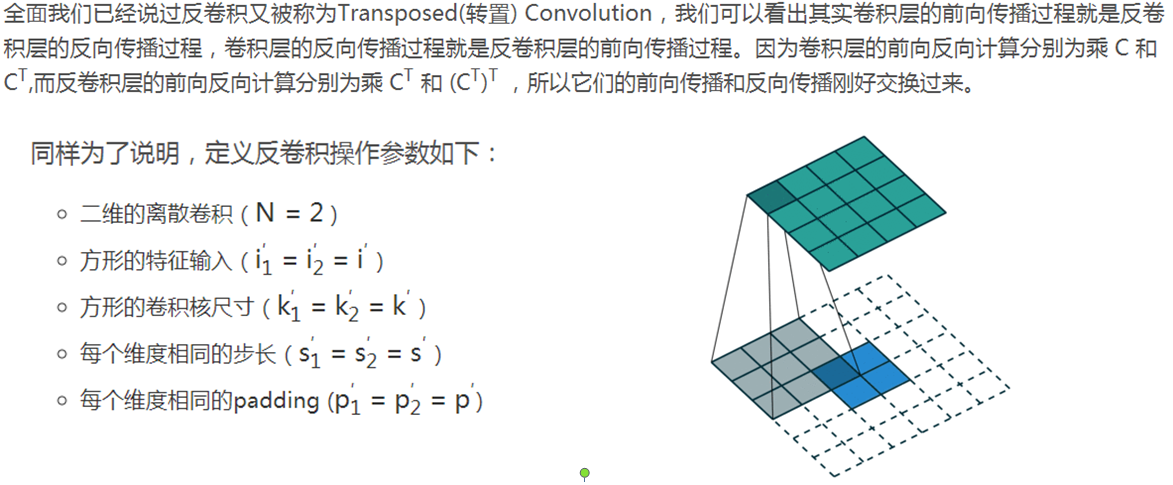$$o′=i′-k′+2p′+1=i′+(k-1)-2p$$

GAN的优点
●GAN是一种生成式模型，相比较其他生成模型（玻尔兹曼机和GSNs）只用到了反向传播
●相比其他所有模型, GAN可以产生更加清晰，真实的样本
●GAN采用的是一种无监督的学习方式训练，可以被广泛用在无监督学习和半监督学习领域

GAN的缺点
●训练GAN需要达到纳什均衡,有时候可以用梯度下降法做到,有时候做不到.我们还没有找到很好的达到纳什均衡的方法,所以训练GAN相比VAE或者PixelRNN是不稳定的
●GAN不适合处理离散形式的数据，比如文本
●GAN存在训练不稳定、梯度消失、模式崩溃的问题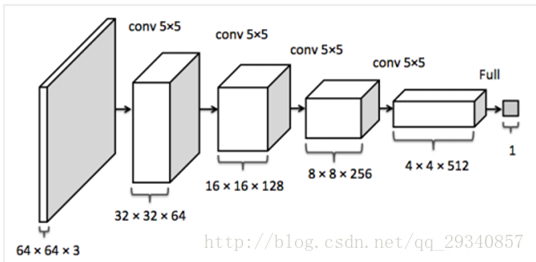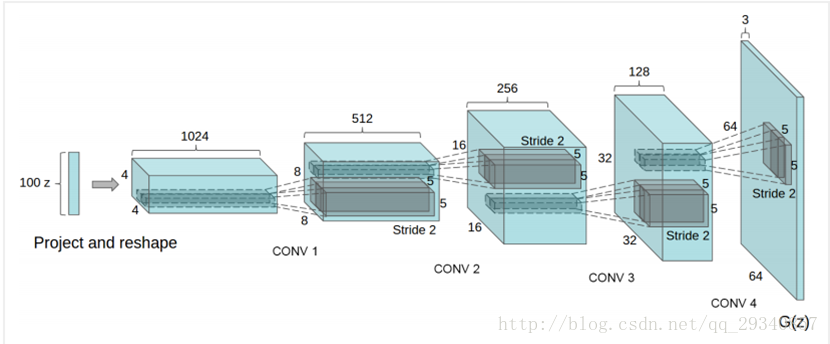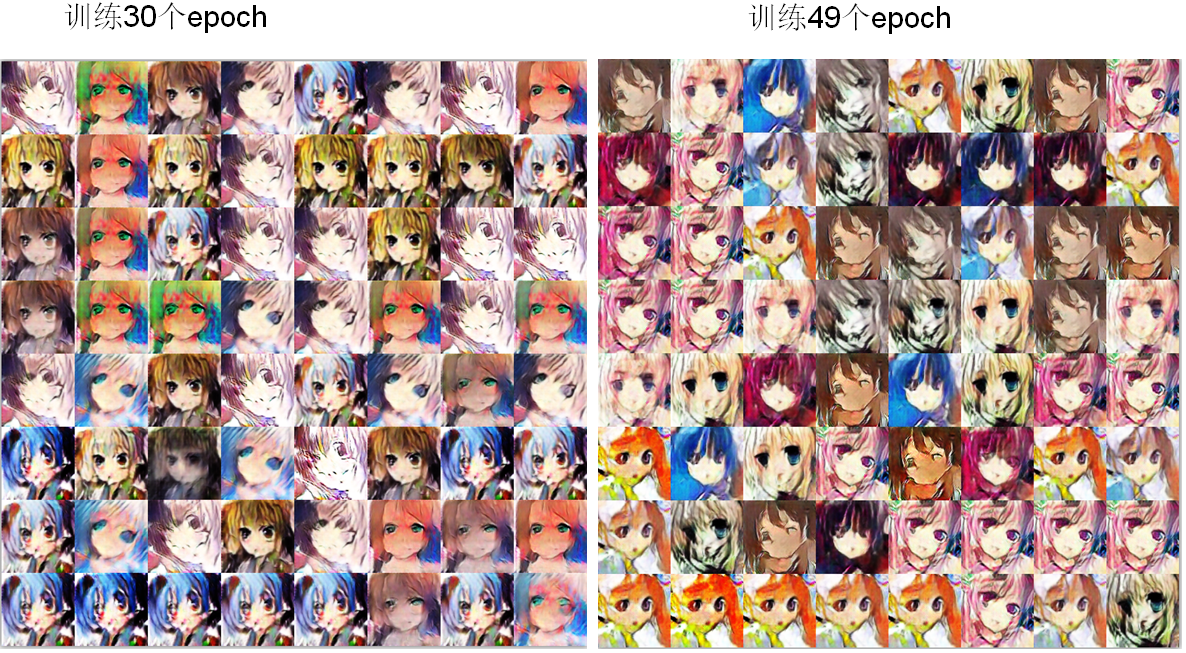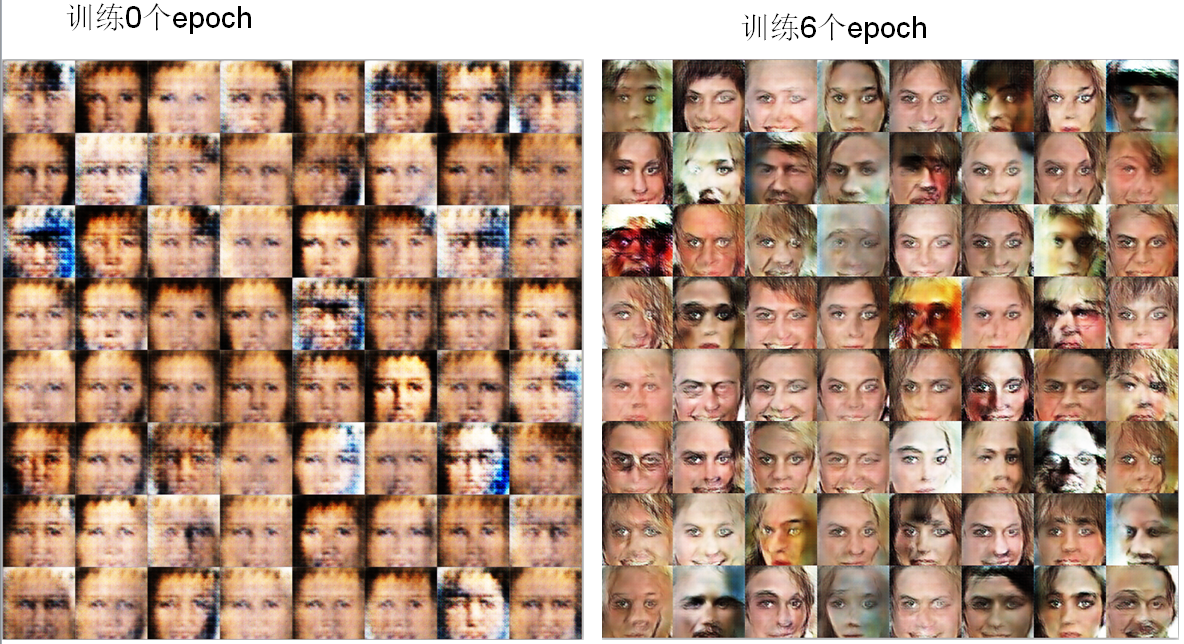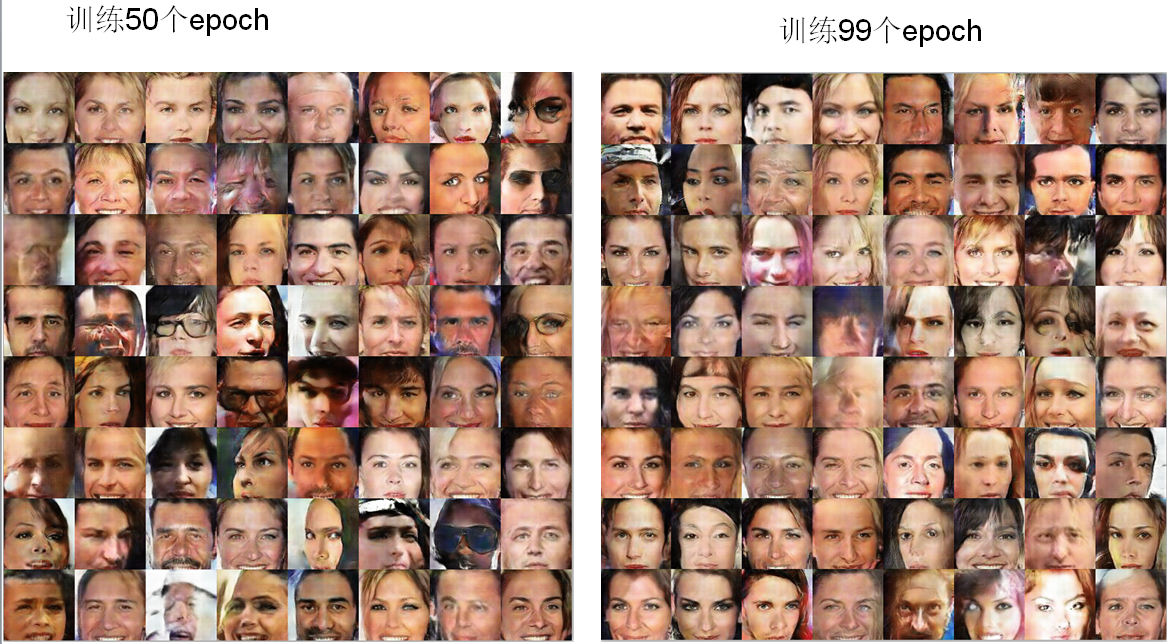------ 本文结束------